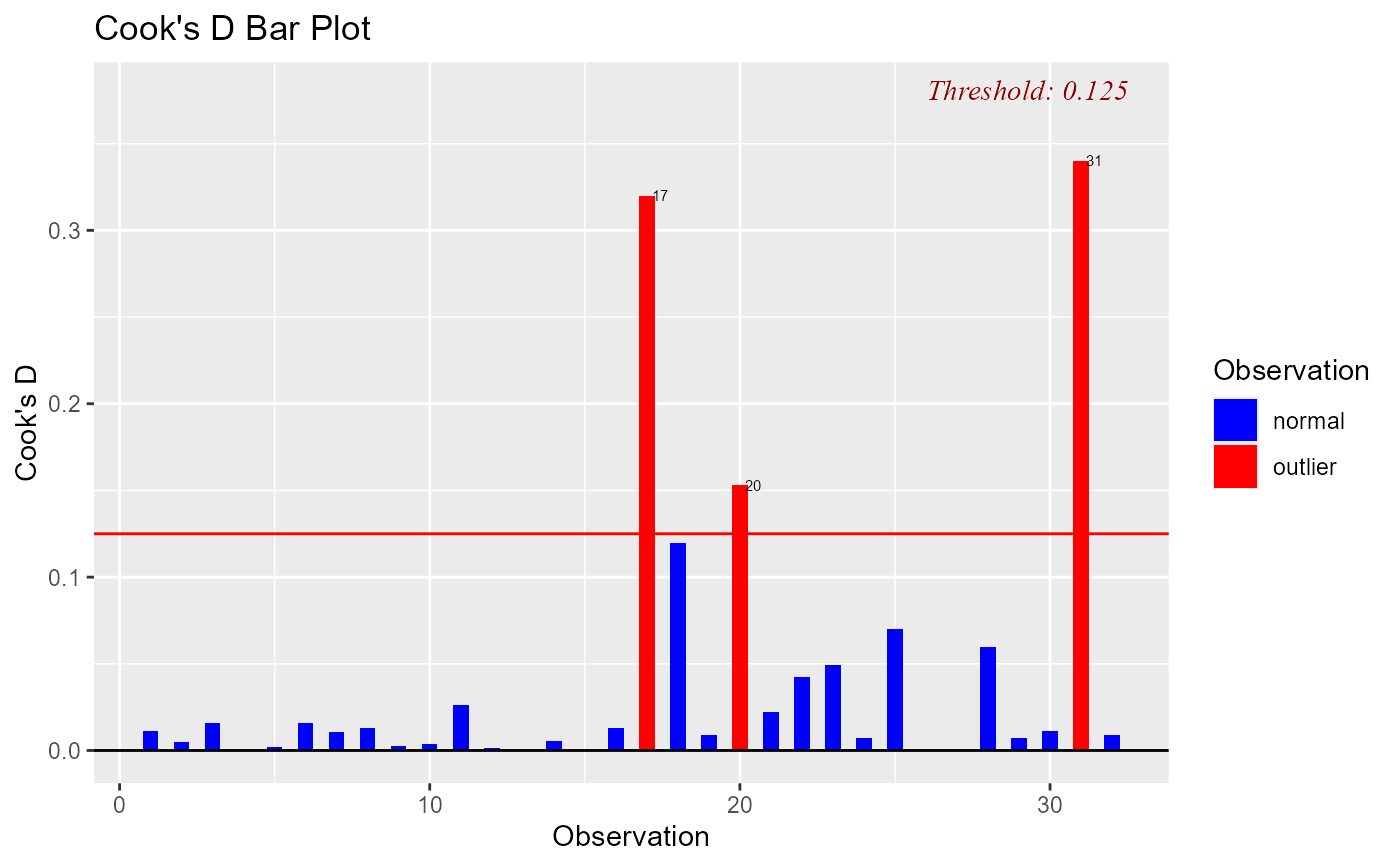Bar Plot of cook's distance to detect observations that strongly influence fitted values of the model.

ols_plot_cooksd_bar(model, type = 1, print_plot = TRUE)

## Arguments

model An object of class lm. An integer between 1 and 5 selecting one of the 6 methods for computing the threshold. logical; if TRUE, prints the plot else returns a plot object.

## Value

ols_plot_cooksd_bar returns a list containing the following components:

outliers

a data.frame with observation number and cooks distance that exceed threshold

threshold

threshold for classifying an observation as an outlier

## Details

Cook's distance was introduced by American statistician R Dennis Cook in 1977. It is used to identify influential data points. It depends on both the residual and leverage i.e it takes it account both the x value and y value of the observation.

Steps to compute Cook's distance:

• Delete observations one at a time.

• Refit the regression model on remaining $$n - 1$$ observations

• examine how much all of the fitted values change when the ith observation is deleted.

A data point having a large cook's d indicates that the data point strongly influences the fitted values. There are several methods/formulas to compute the threshold used for detecting or classifying observations as outliers and we list them below.

• Type 1 : 4 / n

• Type 2 : 4 / (n - k - 1)

• Type 3 : ~1

• Type 4 : 1 / (n - k - 1)

• Type 5 : 3 * mean(Vector of cook's distance values)

where n and k stand for

• n: Number of observations

• k: Number of predictors

## Deprecated Function

ols_cooksd_barplot() has been deprecated. Instead use ols_plot_cooksd_bar().

model <- lm(mpg ~ disp + hp + wt, data = mtcars)